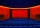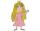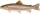# Voting

400 employees cast their votes in a board member election that has only 2 candidates. 120 people vote for candidate A, while half of the remaining voters abstain. How many votes does candidate B receive?

Correct result:

B =  140

#### Solution:We would be pleased if you find an error in the word problem, spelling mistakes, or inaccuracies and send it to us. Thank you!## Next similar math problems:

• Students at cinemaAll pupils from the school went to the cinema for a film performance. The cinema has 400 seats, 2 rows of 20 seats remain unoccupied. 10 places were occupied by teachers. How many students were there?
• PrincessPrincess has lost 321 beads and Prince George are all trying to find. On the first day he found all but 83 beads. On the second day he found 68 beads. On the third day he found the rest, but the princess had lost 76. How many beads had Princess after the
• Flood waterFlood waters in some US village meant that the homes had to evacuate 280 people. 50 of them stayed at elementary schools, 69 them slept with their friends and others went to relatives. How many people have gone to relatives?
• Temperature variationsToday's temperature was 80 degrees, and then the temperature dropped 10 degrees. Then it dropped 15 degrees again, then the next day, the temperature went up 2 degrees. What would the temperature be?
• A troutA trout was swimming 9 feet below the surface of a lake. It dove down 8 more feet to search for food. What is the position of the trout now relative to the surface? To solve the problem, Denise subtracted 9–8 and came up with an answer of 17 feet. Is Deni
• I think numberI think number.When I add 841 to it and subtract 157, I get a number that is 22 greater than 996. What number I thinking?
• SubtractionTest what do you know about the subtraction of two numbers: a) make a difference if the minuend 4,307,288 and subtrahend is 472008 b) minuend is 4000 more than subtrahend. What's the difference? c) the difference is 38900 less than the minuend. What is th
• Roman numerals 2-Subtract up the number written in Roman numerals. Write the results as Roman numbers.
• SummandOne of the summands is 145. The second is 10 more. Determine the sum of the summands.
• The number 3Ski organizers should print the start numbers from 1 to 45. How many times will they use the number 3 when printing?
• Expression 1What is 7+8-(5×2)+5-4+(6×(5-3)+6)-(8+10)-7+6?
• What is missing (1000)What number is to add to get 1000?
• Roman numerals 2+Add up the number writtens in Roman numerals. Write the results as a roman numbers.
• Write decimalsWrite in the decimal system the short and advanced form of these numbers: a) four thousand seventy-nine b) five hundred and one thousand six hundred and ten c) nine million twenty-six
• Roman numeralsWrite numbers written in Roman numerals as decimal.
• Roman numerals +Add up the number writtens in Roman numerals. Write the results as a decimal number.Added together and write as decimal number: LXVII + MLXIV